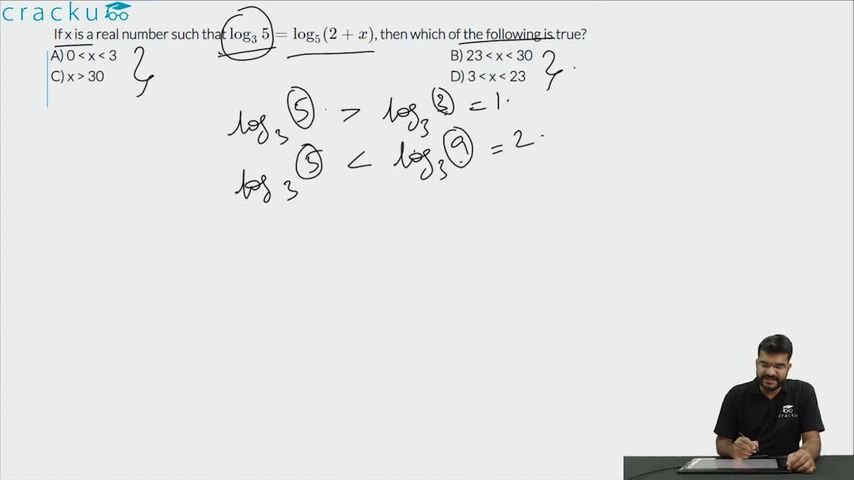Question 88

# If x is a real number such that $$\log_{3}5= \log_{5}(2 + x)$$, then which of the following is true?

Solution

$$1 < \log_{3}5 < 2$$
=> $$1 < \log_{5}(2 + x) < 2$$
=> $$5 < 2 + x < 25$$
=> $$3 < x < 23$$

### View Video Solution• All Quant CAT Formulas and shortcuts PDF
• 30+ CAT previous papers with solutions PDF